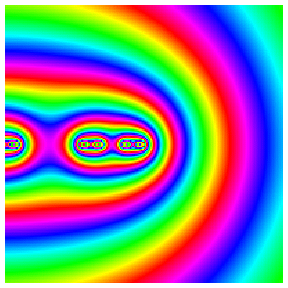# Cantor Potential

The "middle third" Cantor set is a fractal defined by taking a line segment (say between x=-1/2 and x=1/2), removing the middle third, then removing the middle third of the remaining pieces, ad infinitum. It consists of an uncountable number of disconnected points. The animation shows the electrostatic potential of a uniform charge distribution on the Cantor set, zooming into one edge. The colours represent a logarithmic scale, so that identical colours correspond to the factor 3/2 ratio between potentials at equivalent points of the scaling transformation of the fractal, that is a factor 3 in distance. The potential is computed recursively using the equation

V(x,y)=3[V(3x-1,3y)+V(3x+1,3y)]/2

with V approximately given by 1/sqrt(x^2+y^2) at large distances. The relevant paper Potential theory and analytic properties of a Cantor set computes an exact expansion of V(x,y) in horizontal and vertical directions from the end of the fractal, including logarithmic oscillations (too small to observe here) similar to those found in galaxy distributions and the stock market. A recent paper Fractal asymptotics gives references and further applications.

The sound (if you can hear it) is a representation of zooming into the Cantor set, with decreasing pitches a factor of three apart.